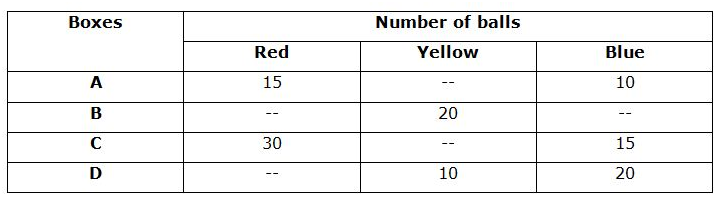# SBI Clerk Prelims 2021 Quantitative Aptitude Questions (Day-48)

Dear Aspirants, Our IBPS Guide team is providing new series of Quantitative Aptitude Questions for SBI Clerk Prelims 2020 so the aspirants can practice it on a daily basis. These questions are framed by our skilled experts after understanding your needs thoroughly. Aspirants can practice these new series questions daily to familiarize with the exact exam pattern and make your preparation effective.

Start Quiz

Table chart

Directions (01-05): Study the following information carefully and answer the given questions:

The given table shows the number of three different color balls in four different boxes.1) The average number of blue balls in all the boxes together is 15 and the number of blue balls in B is equal the number of red balls in box D. What is the average number of balls in D?

A.20

B.10

C.15

D.12

E.None of these

2) Ratio of the number of red balls in B and D is 2:1 and average number of balls in box B is 25. If the total number of red balls in all the boxes together is 75, what is the number of blue balls in box B?

A.30

B.35

C.20

D.25

E.15

3) Total number of balls in box D is 45 and the ratio of the number of red balls in D to the number of yellow balls in A is 3:4. What is the total number of balls in A?

A.40

B.30

C.35

D.45

E.None of these

4) Average number of balls in C is 21 and the average number of yellow balls in all the boxes together is 15. What is the ratio of the number of yellow balls in A to the number of blue balls in D?

A.2:3

B.3:5

C.4:7

D.1:3

E.5:8

5) Total number of balls in all the boxes together is 200. Ratio of the total number of balls in A, B, C and D is 9:9:13:9 and ratio of the red and blue balls in B is 2:3. What is the ratio of the number of yellow balls in A and C together to the number of red balls in B and D together?

A.4:3

B.5:3

C.8:5

D.7:3

E.None of these

Simplification

Directions (06-10): What value should come in place of question mark in the following questions?

6) 23 * √16 + 540 ÷ √1296 – 27 * 4=?

A.-1

B.-2

C.-3

D.-4

E.-5

7) 45% of 160 + 125 % of 80 + ? * 13=157 * √9

A.21

B.23

C.27

D.29

E.31

8) 210 ÷ 7 + ? % of 63=131 * 6 + 189

A.1200

B.1400

C.1300

D.1500

E.1100

9) 25 * 8 – ? + 12 * 4=80 % of 140

A.136

B.132

C.128

D.120

E.124

10) 65% of 120 + 15 * 35 – √841 * 8=?

A.341

B.351

C.361

D.371

E.381

Total number of blue balls=15 * 4=60

Number of blue balls in B=60 – 10 – 15 – 20=15

Average number of balls in D=(10 + 20 + 15)/3=15

Red balls=75

Number of red balls in B and D=75 – 15 – 30=30

Number red balls in B=2/3 * 30=20

Total number of red balls in box B=25 * 3=75

Number of blue balls in B=75 – 20 – 20=35

Number of red balls in D=45 – 10 – 20=15

Number of yellow balls in A=4/3 * 15=20

Total number of balls in A=20 + 15 + 10=45

Total number balls in C=21 * 3=63

Yellow balls in C=63 – 30 – 15=18

Total number of yellow balls=15 * 4=60

Yellow balls in A=60 – 20 – 18 – 10=12

Number of blue balls in D=20

Required ratio=12:20=3:5

Total number of balls in A =9/40 * 200=45

Number of yellow balls in A=45 – 15 – 10=20

Total number of balls in C=13/40 * 200=65

Number of yellow balls in C=65 – 30 – 15=20

Total number balls in B=9/40 * 200=45

Number of red balls in B=2/5 * (45 – 20)=10

Total number of balls in D=9/40 * 200=45

Number of red balls in D=45 – 10 – 20=15

Required ratio=(20 + 20):(10 + 15)

=40:25

=8:5

23 * √16 + 540 ÷ √1296 – 27 * 4=?

92 + 15 – 108=?

-1=?

45% of 160 + 125 % of 80 + ? * 13=157 * √9

72 + 100 + ? * 13=471

? * 13=299

?=23

210 ÷ 7 + ? % of 63=131 * 6 + 189

30 + ? % of 63=975

? % of 63=945

?=1500

25 * 8 – ? + 12 * 4=80 % of 140

200 – ? + 48=112

?=136

65% of 120 + 15 * 35 – √841 * 8=?

78 + 525 – 232=?

?=371

 Check Here to View SBI Clerk Prelims 2021 Quantitative Aptitude Questions Day – 47 Day – 46 Day – 45